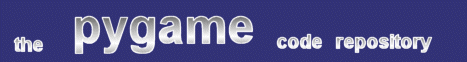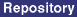### Title: Aspect Scale

Author: Frank Raiser (crashchaos at gmx.net)
Submission date: September 6, 2001

Description: Scaling surfaces keeping their aspect ratio

Download: aspect_scale.py

pygame version required: 1.1
SDL version required: Any
Python version required: Any

Comments: As Mr. Raiser says, this is a pretty simple and straightforward variation on the standard pygame.transform.scale function. This one will preserve the height/width ratio of your image as it scales it, so that no distortion occurs. As a result, you won't necessarily get the exact final surface dimensions you request.

```
"""
aspect_scale.py - Scaling surfaces keeping their aspect ratio
Raiser, Frank - Sep 6, 2k++
crashchaos at gmx.net

This is a pretty simple and basic function that is a kind of
enhancement to pygame.transform.scale. It scales a surface
(using pygame.transform.scale) but keeps the surface's aspect
ratio intact. So you will not get distorted images after scaling.
A pretty basic functionality indeed but also a pretty useful one.

Usage:
is straightforward.. just create your surface and pass it as
first parameter. Then pass the width and height of the box to
which size your surface shall be scaled as a tuple in the second
parameter. The aspect_scale method will then return you the scaled
surface (which does not neccessarily have the size of the specified
box of course)

Dependency:
a pygame version supporting pygame.transform (pygame-1.1+)
"""

def aspect_scale(img,(bx,by)):
""" Scales 'img' to fit into box bx/by.
This method will retain the original image's aspect ratio """
ix,iy = img.get_size()
if ix > iy:
# fit to width
scale_factor = bx/float(ix)
sy = scale_factor * iy
if sy > by:
scale_factor = by/float(iy)
sx = scale_factor * ix
sy = by
else:
sx = bx
else:
# fit to height
scale_factor = by/float(iy)
sx = scale_factor * ix
if sx > bx:
scale_factor = bx/float(ix)
sx = bx
sy = scale_factor * iy
else:
sy = by

return pygame.transform.scale(img, (sx,sy))

```

 Feedback